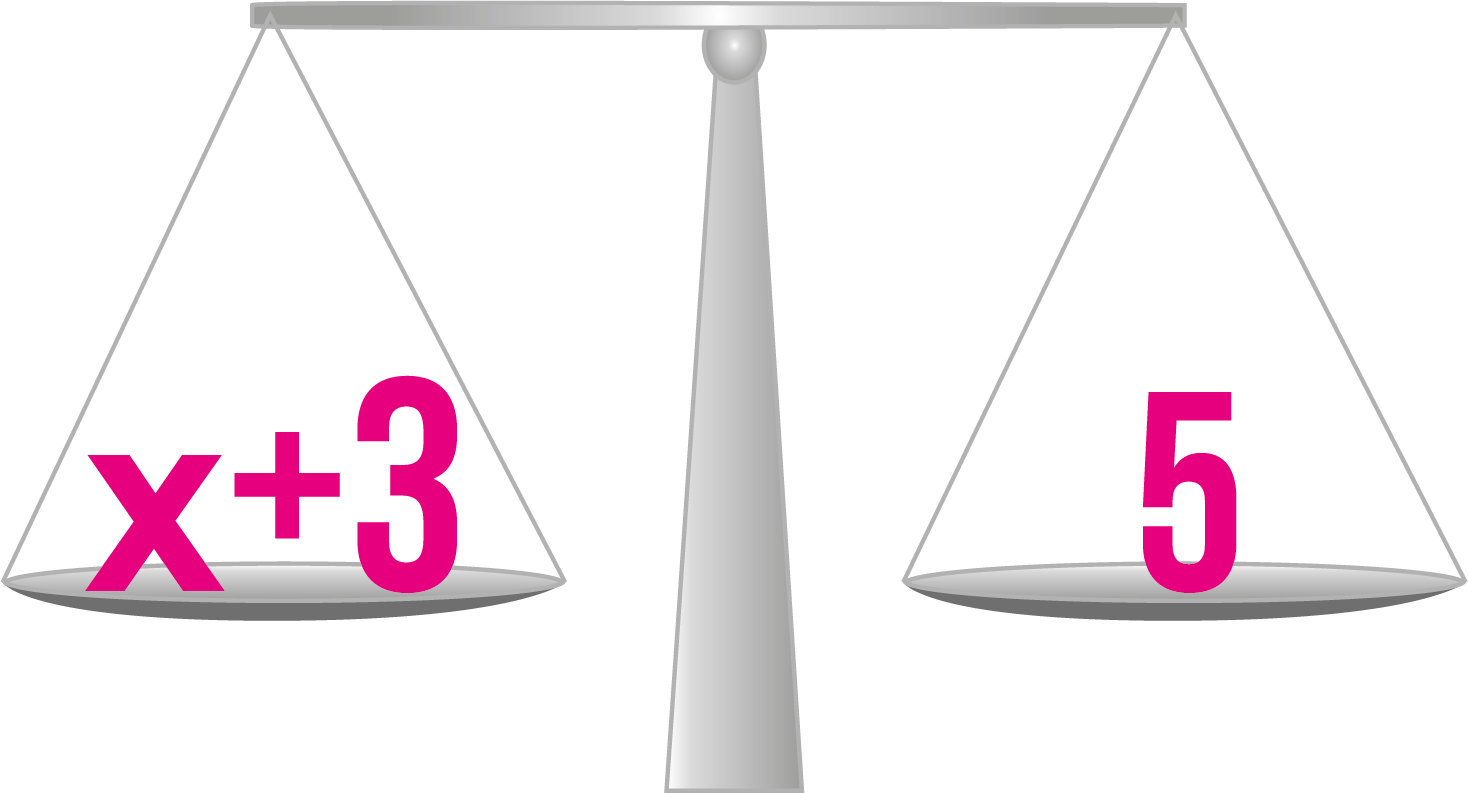# What Is an Equation?

An equation is a mathematical expression with the goal of finding the value of a variable. The variable we use in equations much of the time is $x$. An equation is made up of a left hand side and a right hand side, separated by an equal sign. Here’s an example of an equation:

 $x+3=5$

What number do you have to replace $x$ with to make the left hand side equal to $5$? Or put another way: What do you have to add to $3$ to get $5$?This equation looks really easy. Maybe you’re wondering why you even need to bring a variable into this to find the answer.

The reason is that, as you get more comfortable with equations, the expressions on both sides of the equal sign will grow bigger and more difficult to keep tabs on. When that happens, you might not be able to find the value of $x$ just by thinking about it. That’s why we’ve developed rules to make the process much easier.

When you work with equations, we call it solving equations. What you want to focus on, when you’re trying to find the value of $x$, is to keep the scales above balanced.

What happens to the scales if you switch $x$ with the number 1?

The left scale becomes

 $x+3=1+3=4.$

Because $4$ is less than $5$, the left scale will be lighter than the right scale. Then the right scale will fall towards the ground, and the scales won’t be balanced anymore.

What happens to the scales if you switch $x$ with the number 7?

The left scale becomes

 $x+3=7+3=10.$

Since $10$ is greater than $5$, the left scale will be heavier than the right scale. Then the left scale will fall towards the ground, and the scales won’t be balanced anymore.

What happens to the scales if you switch $x$ with the number 2?

The left scale becomes

 $x+3=2+3=5.$

This means that the scales are balanced when $x=2$! The value of the left hand side is $5$ and the value of the right hand side is $5$. We’ve reached our goal!